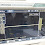# 1000W ICs audio amplifier with PA03

This is a series of amplifiers are based on the most high-ic power that I have ever known. Because the output is issued up to 1000 watts with the impedance RL 4Ohm. Current supply 120mA, and 30A output current. Minimum input voltage of about 30V and a maximum voltage up to 150V. This is an audio amplifier that I have come across use ICs that maximum voltage up to 150V and output power up to 1000W , the scheme below gan.

Part List
Resistor
R1 = 10K
R2 = 10K
R3 = 220K
R4 = 0.18R 5W
R5 = 2.2R 2W
R6 = 0.18R 5W
Capacitor
C1 = 1uF
C2 = 1000uF
C3 = 68pF
C4 = 1000uF
Inductor
L1 = 4MH
IC
U1 = PA03

## 2 comments for "1000W ICs audio amplifier with PA03"

1.where it is available

1.its so difficult to find it.